Homework Help Question & Answers

# 1. Please test hypothesis for a mean or for a proportion. Please state null and alternative...

1. Please test hypothesis for a mean or for a proportion. Please state null and alternative hypothesis

Hypothesized mean = 125, sample mean = 135, standard deviation = 15; n= 500, confidence level = 95%

2. Test Hypotheses of population percent = 33%， sample percent = 31%，n= 120， confidence level = 99%

#### Homework Answers

Answer #1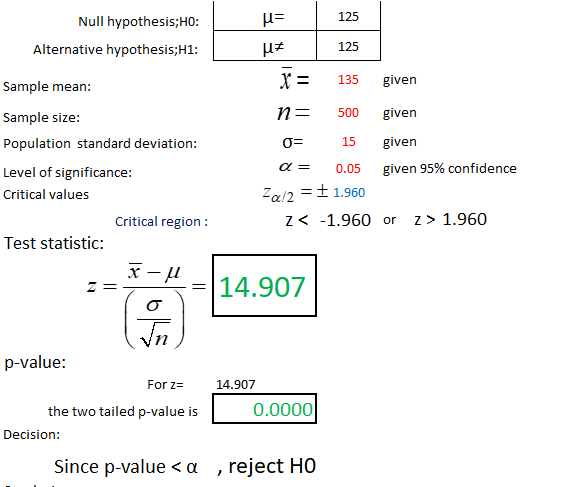This is a test of a single mean

Know the answer?
Your Answer:

#### Post as a guest

Your Name:

What's your source?

#### Earn Coin

Coins can be redeemed for fabulous gifts.

Not the answer you're looking for? Ask your own homework help question. Our experts will answer your question WITHIN MINUTES for Free.
Similar Homework Help Questions
• ### "Find the power of the test, when the Null Hypothesis assumes a population mean of Mu...

"Find the power of the test, when the Null Hypothesis assumes a population mean of Mu = 450, with a populaiion standard deviation of 156, the sample size is 5 and the true mean is 638.47 with confidence itnervals of 95%" "Find the power of the test, when the Null Hypothesis assumes a population mean of Mu = 644, with a populaiion standard deviation of 174, the sample size is 3 and the true mean is 744.04 with confidence itnervals...

• ### "Find the power of the test, when the Null Hypothesis assumes a population mean of Mu...

"Find the power of the test, when the Null Hypothesis assumes a population mean of Mu = 450, with a populaiion standard deviation of 156, the sample size is 5 and the true mean is 638.47 with confidence itnervals of 95%" "Find the power of the test, when the Null Hypothesis assumes a population mean of Mu = 644, with a populaiion standard deviation of 174, the sample size is 3 and the true mean is 744.04 with confidence itnervals...

• ### last option is population proportion The null and alternative hypotheses are given. Determine whether the hypothesis...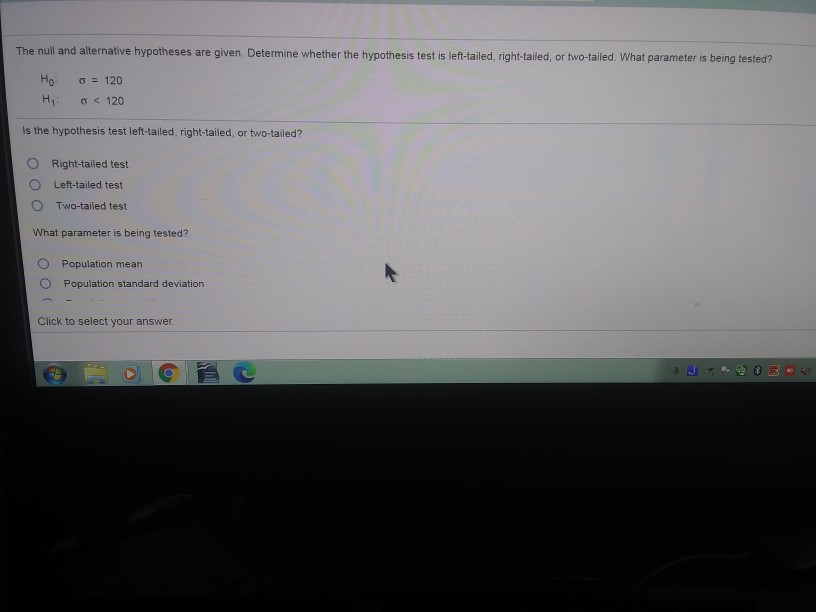last option is population proportion The null and alternative hypotheses are given. Determine whether the hypothesis test is left-tailed, right-tailed, or two-tailed. What parameter is being tested? Ho 0 = 120 Hi 0 < 120 Is the hypothesis test left-tailed, right-tailed, or two-tailed? Right-tailed test Left-tailed test Two-tailed test What parameter is being tested? Population mean Population standard deviation Click to select your answer.

• ### In a test of hypothesis, the null hypothesis is that the population mean is equal to...

In a test of hypothesis, the null hypothesis is that the population mean is equal to 90 and the alternative hypothesis is that the population mean is not equal to 90. The test is to be made at the 10% significance level. A sample of 100 elements selected from this population produced a mean of 84 and a standard deviation of 8. What is the value of the observed test statistic, z?

• ### STEP 2 State the Null and Alternative (Research) hypotheses NULL Hypothesis ALTERNATIVE Hypothesis Is this a...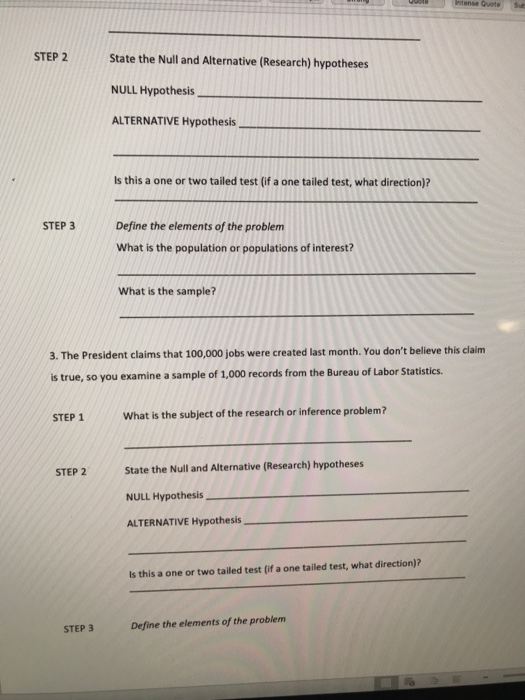STEP 2 State the Null and Alternative (Research) hypotheses NULL Hypothesis ALTERNATIVE Hypothesis Is this a one or two tailed test (if a one tailed test, what direction)? STEP 3 Define the elements of the problem What is the population or populations of interest? What is the sample? 3. The President claims that 100,000 jobs were created last month. You don't believe this claim is true, so you examine a sample of 1,000 records from the Bureau of Labor Statistics...

• ### Hypothesis Problems For the following hypothesis tests: a. State the null (Ho) and alternative (Hi) hypotheses b. State the type of test (right-tailed, left-tailed, or two-tailed) c. State the m...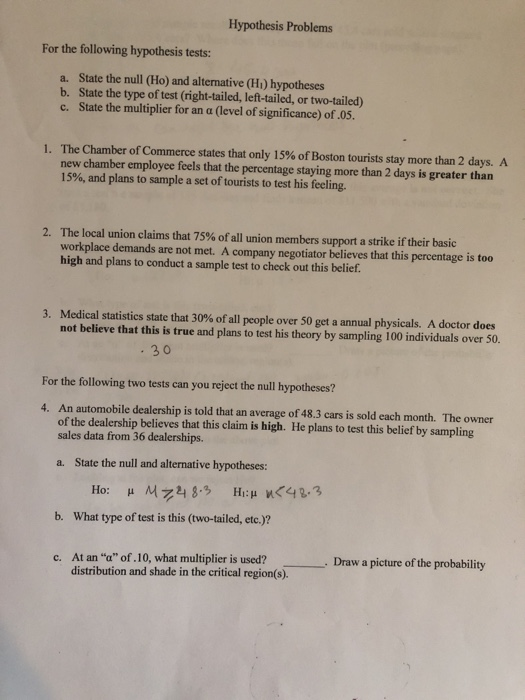Hypothesis Problems For the following hypothesis tests: a. State the null (Ho) and alternative (Hi) hypotheses b. State the type of test (right-tailed, left-tailed, or two-tailed) c. State the multiplier for an a (level of significance) of .05. The Chamber of Commerce states that only 15% of Boston tourists stay more than 2 days. A new chamber employee feels that the percentage staying more than 2 days is greater than 15%, and plans to sample a set of tourists to...

• ### Step 1 of 4: State the null and alternative hypotheses for the test. Step 2 of...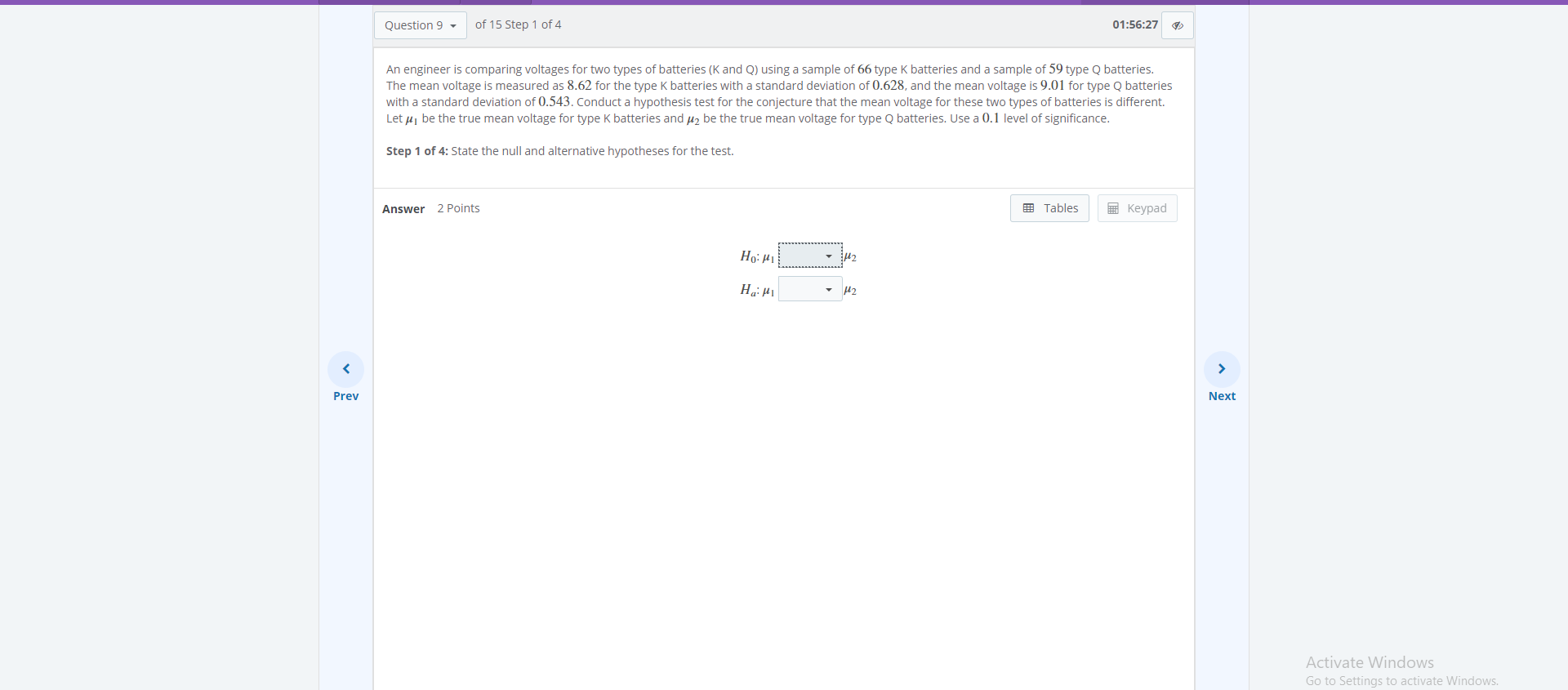Step 1 of 4: State the null and alternative hypotheses for the test. Step 2 of 4: Compute the value of the test statistic. Round your answer to two decimal places. Step 3 of 4: Determine the decision rule for rejecting the null hypothesis H0H0. Round the numerical portion of your answer to three decimal places. Step 4 of 4: Make the decision for the hypothesis test. Question 9 of 15 Step 1 of 4 01:56:27 An engineer is comparing...

• ### 3. Testing a population mean The test statistic (Chapter 11) Aa Aa You conduct a hypothesis...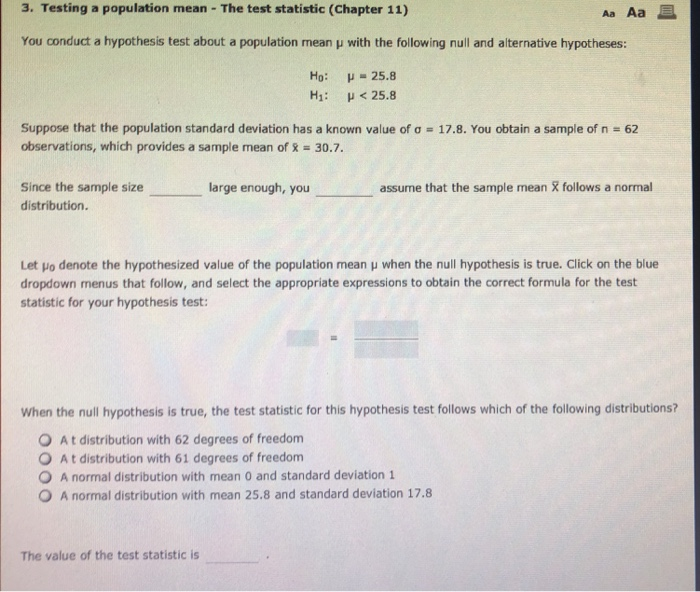3. Testing a population mean The test statistic (Chapter 11) Aa Aa You conduct a hypothesis test about a population mean u with the following null and alternative hypotheses: Ho: u-25.8 H1: <25.8 Suppose that the population standard deviation has a known value of a observations, which provides a sample mean of % 30.7. 17.8. You obtain a sample of n =62 Since the sample size large enough, you assume that the sample mean X follows a normal distribution. Let...

• ### 3) A power company wished to test the null hypothesis that the mean time for processing...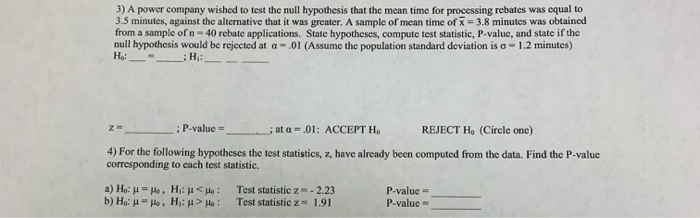3) A power company wished to test the null hypothesis that the mean time for processing rebates was equal to 3.5 minutes, against the alternative that it was greater. A sample of mean time of 3.8 minutes was obtained from a sample of n - 40 rebate applications. State hypotheses, compute test statistic, P-value, and state if the null hypothesis would be rejected at -.01 (Assume the population standard deviation is -1.2 minutes) H : - H: : P-value ;...

• ### Test the given claim. Identify the null​ hypothesis, alternative​ hypothesis, test​ statistic, P-value, and then state...

Test the given claim. Identify the null​ hypothesis, alternative​ hypothesis, test​ statistic, P-value, and then state the conclusion about the null​ hypothesis, as well as the final conclusion that addresses the original claim. Among 2031 passenger cars in a particular​ region, 227 had only rear license plates. Among 300 commercial​ trucks, 45 had only rear license plates. A reasonable hypothesis is that commercial trucks owners violate laws requiring front license plates at a higher rate than owners of passenger cars....

Free Homework App

Scan Your Homework
to Get Instant Free Answers
Need Online Homework Help?

Get Answers For Free
Most questions answered within 3 hours.